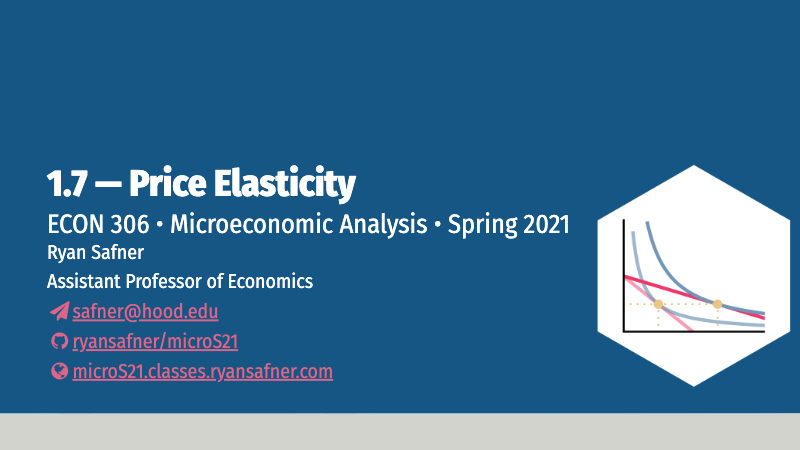1.7 — Price Elasticity — Class Content

Contents

• Section 1: Monday, February 22, 2021
• Section 2: Thursday, February 25, 2021Section 2, because of no class on Thursday Feb 18, is a class behind.

Overview

Today we wrap up Unit 1 by talking more extensively about demand curves and simple demand functions (relating price and quantity demanded of a good, ceterus paribus). We also (re-)introduce the idea of price elasticity of demand that measures a person’s responsiveness in consumption to a change in that good’s price. However, unlike what you learned in principlesArc-price elasticity using the midpoint between two points on the demand curve: $\cfrac{\frac{q_{2}-q_{1}}{\left(\frac{q_{1}+q_{2}}{2}\right)}}{\frac{p_{2}-p_{1}}{\left(\frac{p_{1}+p_{2}}{2}\right)}}$ This actually calculates the elasticity of a single point, the one midway between $$(q_1,p_1)$$ and $$(q_2,p_2)$$.

we look at the elasticity of any point on the demand curve using a more useful formula: $\epsilon_{q,p}=\frac{1}{slope} \times \frac{p}{q}$ where slope refers to the slope of the inverse demand curve (the one that we graph).

We will look at the key relationship between price elasticity of demand and total revenue,Total expenditure, from the perspective of the buyer.

and work on some practice problems.

To allow you to work on Exam 1 (see below), there will be no class on Monday Mar 1 (for section 1 M/W) or Thursday Mar 4 (for section 2 Tu/Th).

• Ch. 2.2, 2.5, 5.5, in Goolsbee, Levitt, and Syverson, 2019

SlidesPractice

Today we will be working on practice problems. Answers will be posted later on that page.

Example Applications of Consumer Theory Models

1. Uncertainty: We have examined cases where outcomes are certain. What if outcomes are not guaranteed, but are uncertain (i.e. probabilistic)? Things like your health, your investments, and the integrity of your property (from theft, natural disasters, war, etc) certainly fall into this category. For this reason, we take out insurance on valuable things that have some probability of significantly losing their value. Let’s explore how Consumer Theory can make our actions more intelligible.
2. Exchange: We have examined the case of a single individual (a consumer) making optimal choices given market prices, income, and their preferences. In one sense, an economy is just a collection of millions of these individuals all doing the same thing. But economics truly begins where at the more important stage where these individuals interact and exchange with one another. In particular, we want to examine how relative prices and income are determined in an economy (up until now we simply assumed them as given parameters). They must come from somewhere! – the interactions of individuals buying and selling with one another, responding in order to balance supply and demand. This is where the study of (general) equilibrium comes from, and what the traditional Supply and Demand model captures in its simplifications.
3. Taxation: This handout uses consumer theory to answer a simple question: which is better for consumers, a consumption tax (tax per unit), or an equivalent income tax that raises the same amount of revenue for the government?
4. Intertemporal Choice (TBD): All of our models thus far have only looked at present consumption and ignored any element of time. We can also look at intertemporal choice, that is, consumption decisions over time. This often touches on topics that most consider “macroeconomics,” concepts like interest rates, borrowing, lending, etc. However, these “big picture” ideas must come from actual individual decisions to consume or to save, aggregated across many individuals. Even macroeconomic theories often require “micro-foundations.” Abstract and complex concepts like interest are actually just applications of relative price theory–interest itself is a relative price of consumption today vs. consumption in the future!